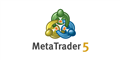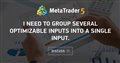# I need to group several optimizable inputs into a single input.

Good night.

I have the following , where I need help.

I have several boolean variables, which I would like to summarize to an int variable in an easier way than the example below, after all there are dozens of variables.

For example:

``` input int varSingle = 0 ; //optimizable parameter

bool var1 = false ;
bool var2 = false ;
bool var3 = false ;

switch ( varSingle )
{
case    3 || 5 || 6 || 7 :
var1= true ;
case    2 || 4 || 5 || 7 :
var2= true ;
case    1 || 4 || 6 || 7 :
var3= true ;
break ;
}```

Being that it would be the equivalent of all the possible possibilities, as in the following table.

varSingle Var1 Var2 Var3
0 0 0 0
1 0 0 1
2 0 1 0
3 1 0 0
4 0 1 1
5 1 1 0
6 1 0 1
7 1 1 1Double Exponential Moving Average - Trend Indicators - Technical Indicators - Price Charts, Technical and Fundamental Analysis - MetaTrader 5 Help
Double Exponential Moving Average Technical Indicator (DEMA) was developed by Patrick Mulloy and published in February 1994 in the "Technical...

Maybe you could do something like this (untested/uncompiled):

```bool lookup[3,8] = { { 0, 0, 0, 1, 0, 1, 1, 1 },
{ 0, 0, 1, 0, 1, 1, 0, 1 },
{ 0, 1, 0, 0, 1, 0, 1, 1 } };

int  varSingle = 0; // Some value 0 to 7

bool
var1 = lookup[ 0, varSingle ],
var2 = lookup[ 1, varSingle ],
var3 = lookup[ 2, varSingle ];```

Marco Antonio Colognese:

varSingle Var1 Var2 Var3
0 0 0 0
1 0 0 1
2 0 1 0
3 1 0 0
4 0 1 1
5 1 1 0
6 1 0 1
7 1 1 1

You need to use a Switch statement not IF,  to use case arguments

```switch (varSingle )
{
case 1:
var1=false;
var2=false;
var3=true;
break;
case 2:
var1=false;
var2=true;
var3=false;
break;
case 3:
var1=true;
var2=false;
var3=false;
break;
case 4:
var1=false;
var2=true;
var3=true;
break;
case 5:
var1=true;
var2=true;
var3=false;
break;
case 6:
var1=true;
var2=false;
var3=true;
break;
case 7:
var1=true;
var2=true;
var3=true;
break;
default:
var1=false;
var2=false;
var3=false;
break;
}
```

Paul Anscombe #:

You need to use a Switch statement not IF,  to use case arguments

Yes. I was wrong when I wrote here. Thanks for letting me know. But the doubt remains, as I would like a more efficient way for many boolean variables.

Marco Antonio Colognese #: Yes. I was wrong when I wrote here. Thanks for letting me know. But the doubt remains, as I would like a more efficient way for many boolean variables.

You seem to have overlooked my post with a suitable option.I need to group several optimizable inputs into a single input.
• 2022.03.11
• www.mql5.com
Good night. I have the following demand , where I need help...

Marco Antonio Colognese #:

Yes. I was wrong when I wrote here. Thanks for letting me know. But the doubt remains, as I would like a more efficient way for many boolean variables.

In that case Fernandos solution should work fine for you

Marco Antonio Colognese: Being that it would be the equivalent of all the possible possibilities, as in the following table.
varSingle Var1 Var2 Var3
0 0 0 0
1 0 0 1
2 0 1 0
3 1 0 0
4 0 1 1
5 1 1 0
6 1 0 1
7 1 1 1
Your decomposition is just binary, except for 3 and 4, and 5 and 6. Is that intentional? If not just mask the bits.
```void decompose(int single, bool& var1, bool& var2, bool& var3){
var3 = single & 1 != 0;
var2 = single & 2 != 0;
var1 = single & 4 != 0;
}```

Otherwise the lookup table is likely the best.

Fernando Carreiro #:

Maybe you could do something like this (untested/uncompiled):

Your solution so far is the most viable. However, in a case with 10 or more variables it would be complicated, as 10 rows and more than 500 columns would be needed.

William Roeder #:
Your decomposition is just binary, except for 3 and 4, and 5 and 6. Is that intentional? If not just mask the bits.

Otherwise the lookup table is likely the best.

I don't know if I understand your question as I'm using google translator! 😅

But they're all boolean variables, so they're all binary.

And I need all possibilities, from all variables off, to all on.

In the example I posted, I put 3 variables to exemplify, which I meant, so serial only 8 possibilities, (2^3) ("binary variable" ^ "three variables").

But if it is, for example, 10 binary variables, there would be 1024 possibilities.

Can you explain your suggestion better?

Marco Antonio Colognese #: Your solution so far is the most viable. However, in a case with 10 or more variables it would be complicated, as 10 rows and more than 500 columns would be needed.

Then I would suggest you look for the binary patterns so as to convert them into binary bit masking and/or shifting, just as William suggested in his post.

If however, there is no discernable pattern that can be converted, then my option of using a lookup table is still the most viable (and also the fastest).

EDIT: You can also load the lookup table from an external data file as well.I need to group several optimizable inputs into a single input.
• 2022.03.11
• www.mql5.com
Good night. I have the following demand , where I need help...

I found the suggestion of converting from decimal to binary interesting, with the number of digits referring to the number of variables.
So with 10 variables:
1 = 0000000001
2 = 0000000010
3 = 0000000011
.
.
.
1023 = 1111111110
1024 = 1111111111

But how to make this conversion?

After normalizing so that the number of digits is equivalent to the number of variables,

then extracting the digit equivalent to the respective variable,

and finally transforming this information into boolean, is beyond my knowledge. 😅

Reason: